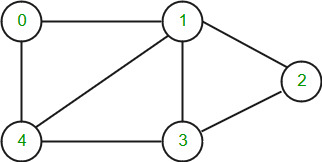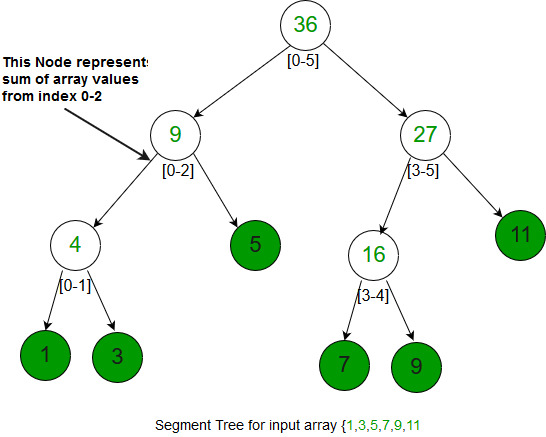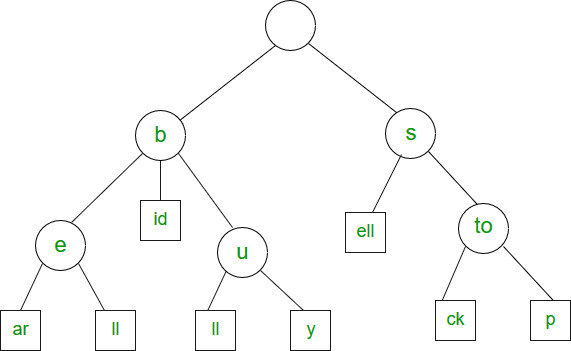Related Articles
Overview of Data Structures | Set 3 (Graph, Trie, Segment Tree and Suffix Tree)
• Difficulty Level : Hard
• Last Updated : 22 Apr, 2020

We have discussed below data structures in previous two sets.

Graph

Graph is a data structure that consists of following two components:

1. A finite set of vertices also called as nodes.
2. A finite set of ordered pair of the form (u, v) called as edge. The pair is ordered because (u, v) is not same as (v, u) in case of directed graph(di-graph). The pair of form (u, v) indicates that there is an edge from vertex u to vertex v. The edges may contain weight/value/cost.

V -> Number of Vertices.
E -> Number of Edges.

Graph can be classified on the basis of many things, below are the two most common classifications :

1. Direction :
Undirected Graph : The graph in which all the edges are bidirectional.Directed Graph : The graph in which all the edges are unidirectional.
2. Weight :
Weighted Graph : The Graph in which weight is associated with the edges.Unweighted Graph : The Graph in which their is no weight associated to the edges.

Graph can be represented in many ways, below are the two most common representations :

Let us take below example graph two see two representations of graph.```Time Complexities in case of Adjacency Matrix :
Traversal :(By BFS or DFS) O(V^2)
Space : O(V^2)

Time Complexities in case of Adjacency List :
Traversal :(By BFS or DFS) O(V + E)
Space : O(V+E)```

Examples : The most common example of the graph is to find shortest path in any network. Used in google maps or bing. Another common use application of graph are social networking websites where the friend suggestion depends on number of intermediate suggestions and other things.

Trie

Trie is an efficient data structure for searching words in dictionaries, search complexity with Trie is linear in terms of word (or key) length to be searched. If we store keys in binary search tree, a well balanced BST will need time proportional to M * log N, where M is maximum string length and N is number of keys in tree. Using trie, we can search the key in O(M) time. So it is much faster than BST.

Hashing also provides word search in O(n) time on average. But the advantages of Trie are there are no collisions (like hashing) so worst case time complexity is O(n). Also, the most important thing is Prefix Search. With Trie, we can find all words beginning with a prefix (This is not possible with Hashing). The only problem with Tries is they require a lot of extra space. Tries are also known as radix tree or prefix tree.

```The Trie structure can be defined as follows :
struct trie_node
{
int value; /* Used to mark leaf nodes */
trie_node_t *children[ALPHABET_SIZE];
};

root
/   \    \
t   a     b
|   |     |
h   n     y
|   |  \  |
e   s  y  e
/  |   |
i  r   w
|  |   |
r  e   e
|
r

The leaf nodes are in blue.

Insert time : O(M) where M is the length of the string.
Search time : O(M) where M is the length of the string.
Space : O(ALPHABET_SIZE * M * N) where N is number of
keys in trie, ALPHABET_SIZE is 26 if we are
only considering upper case Latin characters.
Deletion time : O(M)
```

Example : The most common use of Tries is to implement dictionaries due to prefix search capability. Tries are also well suited for implementing approximate matching algorithms, including those used in spell checking. It is also used for searching Contact from Mobile Contact list OR Phone Directory.

Segment Tree

This data structure is usually implemented when there are a lot of queries on a set of values. These queries involve minimum, maximum, sum, .. etc on a input range of given set. Queries also involve updation of values in given set. Segment Trees are implemented using array.```Construction of segment tree : O(N)
Query : O(log N)
Update : O(log N)
Space : O(N) [Exact space = 2*N-1]```

Example : It is used when we need to find Maximum/Minumum/Sum/Product of numbers in a range.

Suffix Tree

Suffix Tree is mainly used to search a pattern in a text. The idea is to preprocess the text so that search operation can be done in time linear in terms of pattern length. The pattern searching algorithms like KMP, Z, etc take time proportional to text length. This is really a great improvement because length of pattern is generally much smaller than text.
Imagine we have stored complete work of William Shakespeare and preprocessed it. You can search any string in the complete work in time just proportional to length of the pattern. But using Suffix Tree may not be a good idea when text changes frequently like text editor, etc.

Suffix Tree is compressed trie of all suffixes, so following are very abstract steps to build a suffix tree from given text.
1) Generate all suffixes of given text.
2) Consider all suffixes as individual words and build a compressed trie.Example : Used to find find all occurrences of the pattern in string. It is also used to find the longest repeated substring (when text doesn’t change often), the longest common substring and the longest palindrome in a string.